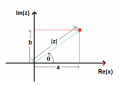# Phase Voltage across an inductor and capacitor

Thread Starter

#### KevinEamon

Joined Apr 9, 2017
281
Hi guys
I'm working on few complex numbers / phasors and all that fun stuff. Learned a lot, it's been good.
Working on this problem.

I come to calculate
VXL = ZLI (I have this in my notes)

= j20 * 2.91∠14.04

= 20 ∠ 90 * 2.91 ∠14.04

= 58.2 ∠ 104.04V

Where the heck is this ∠90 coming from?

Similarly I have ∠-30 when I come to calculate VxC ???

#### WBahn

Joined Mar 31, 2012
26,398
The attachment is being sloppy in its notation, at least by conventional terms.

X, in this context, is generally used to refer to reactance, which is a real number (positive for inductors and negative for capacitors).

The IMPEDANCE of an inductor is Z_L = jωL = jX_L where X_L = ωL is the reactance.

The 90° comes from the representation of j in polar terms, namely 1∠90°.

The impedance angle of a capacitor is -90°. I have no idea where you are getting -30° from.

Thread Starter

#### KevinEamon

Joined Apr 9, 2017
281
Hmmm lil confused...So that would be like two different things.... What I mean is to calculate the inductor we'd be using the representation of j =1∠90°

Is this the impedance of an inductor?

#### WBahn

Joined Mar 31, 2012
26,398
Hmmm lil confused...So that would be like two different things.... What I mean is to calculate the inductor we'd be using the representation of j =1∠90°

Is this the impedance of an inductor?
No, it is the value of j (or √-1) written in polar form.

A complex number is the sum of two parts, a real part and an imaginary part.

z = a + jb

This is the rectangular, or Cartesian, for a complex number.

But we can plot a complex number as a point on the "complex plane" in which the x-axis is the real part and the y-axis is the imaginary part.If a=0 and b=1, we have z = 0 + j1 = j.

The magnitude of the vector is then 1 and the angle is 90° or 1∠90°.

These are both just equivalent ways to represent a particular complex number, namely j.

#### MrAl

Joined Jun 17, 2014
8,259
Hi,

To add a little here...

Mathematically you can calculate the phase angle with the function "atan2(i,r)" where 'r' is the real part and 'i' is the imaginary part. So for an inductor of value 3 Henries we have:
Z=a+b*j=0+L*j=0+3*j=3*j

This has imaginary part (b) equal to 3 and real part (a) equal to zero (0). To find the angle, use:
atan2(3,0)

and it will come out to pi/2 rads which is 90 degrees.

You may want to note that whatever value inductor we have it always becomes the imaginary part in this way and also the real part is always zero, so we always have something like:
atan2(3,0)
atan2(4,0)
atan2(23,0)
atan2(9.234,0)
etc.
and because the real part is zero and the imaginary part is positive, we always get 90 degrees. So with any inductor we always get 90 degrees. Usually we end up calculating the voltage or current though.

There are other ways to do this, namely use atan() instead of atan2() but then there are other things we have to remember with that.

Also, although most people will calculate the principle angle there are times when people want to keep track of the cycles as well. In that rare case you have to also figure out how many full cycles have passed as well as the main phase angle, so that you get the full 'angle' which would then be greater than 360 degrees. This is rare though as usually in AC analysis a phase angle of anything over 360 degrees is considered the same as that angle minus 360, so say 370 degrees would be considered as 10 degrees in most cases because 360 degrees is usually considered to be 0 degrees as is any integer multiple of 360 degrees. 730 degrees would normally be taken as 10 degrees also, etc., etc.

Later when you start to combine the inductor with other components like a resistor this function becomes very handy. A resistor R and inductor L in series become:
Z=R+L*j

with real part R and imag part L, so we have:
angle=atan2(R,L) {usually in rads}

To calculate the current you would first divide the voltage by Z:
I=E/Z=E/(R+L*j)

and then multiply top and bottom by R-L*j (note sign change) and get:
i=E*(R+L*j)/(R^2-j^2*L^2)

and since j^2=-1 we simplify to:
i=E*(R+L*j)/(R^2-(-1)*L^2)=E*(R+L*j)/(R^2+L^2)

and then expand the numerator to get:
i=(E*R+E*L*j)/(R^2+L^2)

and expand more to get:
i=E*R/(R^2+L^2)+E*L*j/(R^2+L^2)

and now this is in the form realpart+imagpart so we can get the phase angle:
angle=atan2(E*R/(R^2+L^2),E*L/(R^2+L^2))

and again that is usually in rads so to convert to degrees we multiply that angle by 180/pi.

Last edited: